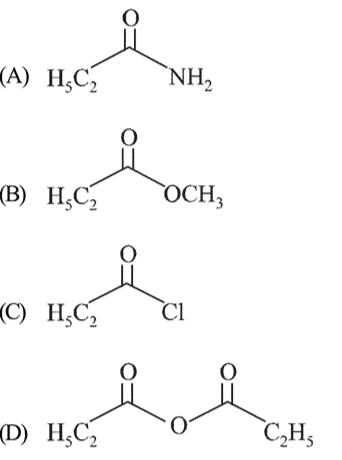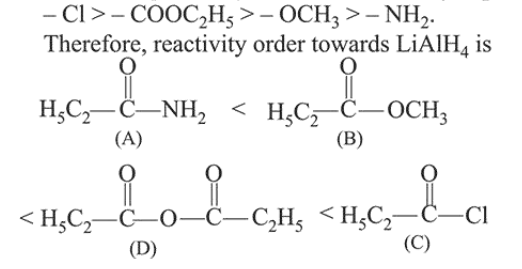# The increasing order of the reactivity of the following

Question:

The increasing order of the reactivity of the following with $\mathrm{LiAlH}_{4}$ is :1. $(B)<(A)<(C)<(D)$

2. $(B)<(A)<(D)<(C)$

3. $(\mathrm{A})<(\mathrm{B})<(\mathrm{D})<(\mathrm{C})$

4. $(A)<(B)<(C)<(D)$

Correct Option: , 3

Solution:

The reactivity order of carboxylic acid derivatives depends on the electrophilicity of the carbonyl carbon and Leaving ability of the four groups is